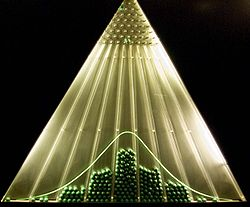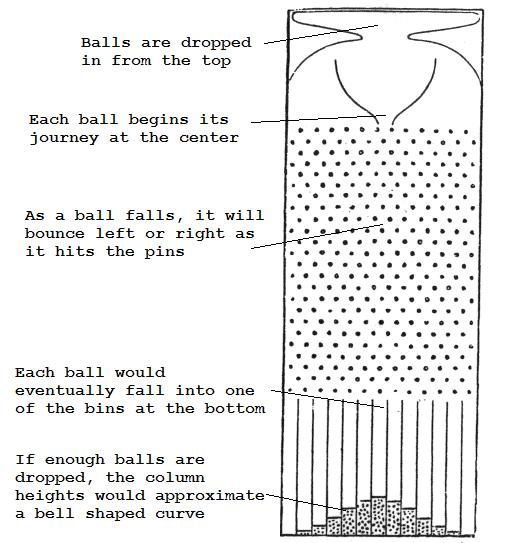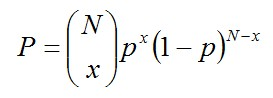# The Galton Box

History of Statistics, History of Science and TechnologyThe Galton Box (also known as a bean machine or quincunx), was invented by Sir Francis Galton in the 19th century.  Its purpose is to demonstrate the central limit theorem and the binomial and normal distributions.As a ball falls, it will bounce either left or right when it hits a pin.  If there are N rows of pins, then the ball would bounce N times.  The position of the bin the ball falls in, would have a binomial distribution where:where:
• x is the bin position e.g. x=0 could be treated as the left-most bin, and x=N could be treated as the right-most bin.
• P is the probability of x
• p is the probability of bouncing right (if x=0 represents the left-most bin).   In an unbiased machine: p = 0.5.
• N is the number of rows of pins i.e. the number of times a ball bounces.
Note that for the positions to conform to a binomial distribution, the number of bins should equal N+1.

If the number of rows of pins is large enough, this would approximate a normal distribution due to the central limit theorem.